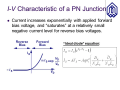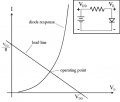# I need your help Pls.To calculate the current in this circuit

#### wtmma

Joined Dec 21, 2012
11
Hello,
I am in the first steps of learning electronics as a hobby, but I always encounter a lot of confusing things that need to be explained. For example, in a simple electronic circuit that contains a resistance (300 ohms), a LED, and a 9-volt battery. To calculate the current in this circuit according to Ohm's law. I =V/R, I = 9/300 = 0,03 mA. But when making measurements on the circuit, the value of the current is slightly different from the result of the calculation. What I want to know is that the reason for the difference is because we did't take in account the resistance value of the LED? In real circuits analysis we have to take into account the resistance value of electronic components (other than resistors) when calculating the value of the current,? and how is this done in practice?
Walid

#### Irving

Joined Jan 30, 2016
3,190
Hi there and welcome to AAC and the wonderful world of electronics.

You are correct in using Ohm's Law, but you have assumed there is 9v across the 300ohm resistor. But there is a voltage drop across the diode too - its 'forward voltage' or Vf in the datasheet - typically 1.5 - 2v for a red LED at normal brightness (10 - 30mA typically). So the actual voltage across the resistor is:

9 - Vf = 9 - 1.8 = 7.2v​
and therefore the current is 7.2/300 = 24mA as shown by this simulation....

As a beginner, some advice - always read and understand the datasheet of any device you use or plan to use.
Spend time learning and understanding how generic components - both passive (R,L,C) and active (diode, transistor, MOSFET) behave. You'll quickly be able to grasp basic circuit functionality.Last edited:

#### BobTPH

Joined Jun 5, 2013
6,077
Yes, you have to take into account all the components. Different components behave differently, you cannot assign a resistance to the LED because it does nit act like a resistor. So you cannot simply use Ohms law to analyze a circuit. Explaining how you analyzing circuits is way beyond what can be done in a forum post. You need to get an elementary text in basic electronics and study it from the beginning if you way to make progress toward your goal.

#### Irving

Joined Jan 30, 2016
3,190
Explaining how you analyzing circuits is way beyond what can be done in a forum post.
While @BobTPH is correct in a generic "how to analyze circuits" sense - its too broad a subject. But if you have a specific circuit you've tried to analyse and need further clarification or help, then by all means post it here with your efforts so far and we'll be happy to assist.

#### MrChips

Joined Oct 2, 2009
27,679
You have selected a rather complex example for learning how to analyze simple circuits using Ohm's Law.
There is no simple solution and one usually has to make assumptions in order to arrive at an estimated answer.

A diode or LED is a non-linear device and does not follow Ohm's Law. You cannot use the resistance of the diode because it changes with current.
You need to know both the current through the diode and the voltage across the diode. For this you need to know the I-V characteristics of the diode.

1) You can make an assumption that the voltage across the diode is about 1.8V as @Irving did in post #2. This is the quick solution.

2) You can do the numerical analysis if you knew the I-V characteristics of the diode.Again, this requires knowledge of particular parameters of the diode equation, for example, the reverse saturation current, Is.
Furthermore, in order to be more precise, you need to know the ambient temperature of the diode since the forward current varies with temperature. You can quickly see how this becomes much more complex than originally thought.

3) You can find a graphical solution knowing the I-V curve of the diode and applying "load-line" analysis.
From this, you find the operating point (or "Q-point") which is the intersection of the diode I-V curve and the resistor load line.
This gives the same solution as for solving with the diode equation in (2) above.#### wtmma

Joined Dec 21, 2012
11
Hi there and welcome to AAC and the wonderful world of electronics.

You are correct in using Ohm's Law, but you have assumed there is 9v across the 300ohm resistor. But there is a voltage drop across the diode too - its 'forward voltage' or Vf in the datasheet - typically 1.5 - 2v for a red LED at normal brightness (10 - 30mA typically). So the actual voltage across the resistor is:

9 - Vf = 9 - 1.8 = 7.2v​
and therefore the current is 7.2/300 = 24mA as shown by this simulation....

As a beginner, some advice - always read and understand the datasheet of any device you use or plan to use.
Spend time learning and understanding how generic components - both passive (R,L,C) and active (diode, transistor, MOSFET) behave. You'll quickly be able to grasp basic circuit functionality.

View attachment 278214
Thank you very much, Irving, you have given me exactly the answer I wanted. I studied the resistors in series and parallel well, but the confusion that occurs when starting to learn electronics seems to prevent me from knowing the correct answer. Thanks

#### wtmma

Joined Dec 21, 2012
11
Yes, you have to take into account all the components. Different components behave differently, you cannot assign a resistance to the LED because it does nit act like a resistor. So you cannot simply use Ohms law to analyze a circuit. Explaining how you analyzing circuits is way beyond what can be done in a forum post. You need to get an elementary text in basic electronics and study it from the beginning if you way to make progress toward your goal.
Thanks,
Can you Pls. suggest me a good text to start?

#### wtmma

Joined Dec 21, 2012
11
You have selected a rather complex example for learning how to analyze simple circuits using Ohm's Law.
There is no simple solution and one usually has to make assumptions in order to arrive at an estimated answer.

A diode or LED is a non-linear device and does not follow Ohm's Law. You cannot use the resistance of the diode because it changes with current.
You need to know both the current through the diode and the voltage across the diode. For this you need to know the I-V characteristics of the diode.

1) You can make an assumption that the voltage across the diode is about 1.8V as @Irving did in post #2. This is the quick solution.

2) You can do the numerical analysis if you knew the I-V characteristics of the diode.
View attachment 278218

Again, this requires knowledge of particular parameters of the diode equation, for example, the reverse saturation current, Is.
Furthermore, in order to be more precise, you need to know the ambient temperature of the diode since the forward current varies with temperature. You can quickly see how this becomes much more complex than originally thought.

3) You can find a graphical solution knowing the I-V curve of the diode and applying "load-line" analysis.
From this, you find the operating point (or "Q-point") which is the intersection of the diode I-V curve and the resistor load line.
This gives the same solution as for solving with the diode equation in (2) above.

View attachment 278219

#### WBahn

Joined Mar 31, 2012
27,880
Hello,
I am in the first steps of learning electronics as a hobby, but I always encounter a lot of confusing things that need to be explained. For example, in a simple electronic circuit that contains a resistance (300 ohms), a LED, and a 9-volt battery. To calculate the current in this circuit according to Ohm's law. I =V/R, I = 9/300 = 0,03 mA. But when making measurements on the circuit, the value of the current is slightly different from the result of the calculation. What I want to know is that the reason for the difference is because we did't take in account the resistance value of the LED? In real circuits analysis we have to take into account the resistance value of electronic components (other than resistors) when calculating the value of the current,? and how is this done in practice?
•